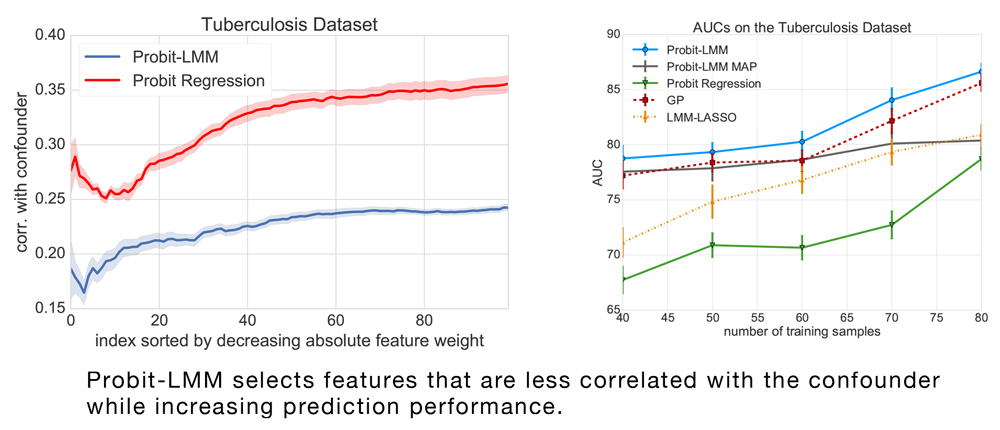# Sparse Probit Linear Mixed Model### Abstract

Linear Mixed Models (LMMs) are important tools in statistical genetics. When used for feature selection, they allow to find a sparse set of genetic traits that best predict a continuous phenotype of interest, while simultaneously correcting for various confounding factors such as age, ethnicity and population structure. Formulated as models for linear regression, LMMs have been restricted to continuous phenotypes. We introduce the Sparse Probit Linear Mixed Model (Probit-LMM), where we generalize the LMM modeling paradigm to binary phenotypes. As a technical challenge, the model no longer possesses a closed-form likelihood function. In this paper, we present a scalable approximate inference algorithm that lets us fit the model to high-dimensional data sets. We show on three real-world examples from different domains that in the setup of binary labels, our algorithm leads to better prediction accuracies and also selects features which show less correlation with the confounding factors.

Type
Publication
Machine Learning, 106(9), 1621-1642
Date